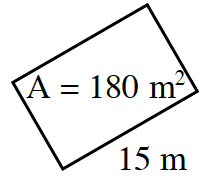### Home > CC3MN > Chapter 1 > Lesson 1.1.4 > Problem1-35

1-35.

The area of each rectangle below is shown in the middle of the rectangle. For each figure, find the missing length or width.

1.  Left edge, blank interior$\text{A} = 60\;\text{in.}^2$ bottom edge $8 \; \text{in.}$

The area of a rectangle is the product of the length and width.

Substitute the area and the unknown
length or the width into the equation
$A=l·w$.

Solve for the unknown variable.

$60=\text{length}\cdot 8$
$\frac{ 60 }{ 8} = \frac{ \text{length }\cdot 8 }{8 }$$\text{length }= \frac{ 60 }{8 } = 7.5$

1.Try not to be confused by the orientation of the rectangle. Solve as in part (a).

1.  interior $\text{A} = 231 \; \text{cm}^2$ right edge$14 \; \text{cm}$ bottom edge, blank

See the example below.

 interior $20 \; \text{cm}^2$ right edge$5$ bottom edge, blank

$20=5\ ·$ length
$\frac{ 20 }{ 5} = \frac{ 5 \cdot \text{length} }{5 }$
$\text{length }= \frac{ 20 }{ 5} = 4$